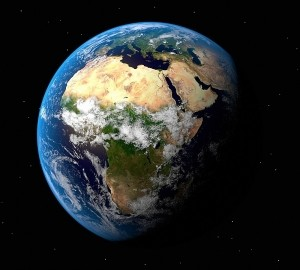•• # What is the Size of Earth?

The size of the Earth in terms of its diameter is 7826 miles at the equatorial level. At the polar level it is 7900 miles (both around 12,800 km). Below are the other important facts and figures about the Earth.

### Earth’s Physical Attributes

The mean radius is 6,371 km and the equatorial radius is 6,378. The polar radius is 6,356 km. The circumference is 40,075 km at the equatorial and 40,007.86 km at the meridian.

The mean is 40,041 km. The total surface area is 510,072,000 sq km. The land area is 148,940,000 sq km and the water area is 361,132,000 km.

The mean density is 5.5153 g/cm3 and the equatorial surface gravity is 9.780327 s/m (square). The size of the Earth volume wise is 9.780327 x 10 (to the 12th power) km3.

The mass is 5.9736 × 1024 kg. The escape velocity has been measured at 11.186 km/s and the equatorial rotation velocity at 1,673 km per hour.### Orbital Statistics

The aphelion is 152,097,701 km (or 1.0167103335 AU). The perihelion is 147,098,074 km (equal to 0.9832898912 AU). The semi major axis is 149,597,887.5 km or 1.0000001124 AU. The eccentricity has been computed to be 0.016710219. The orbital speed is 107,218 km per hour.

### The Earth’s Atmosphere

It is composed of 78.08% nitrogen, 20% oxygen with traces of argon, water vapor and carbon dioxide.

### Land and Crust

The land surface is composed mainly of rocks. The size of the Earth makes it the largest of all the non gaseous planetary bodies in the Solar System. It also possesses the highest density and its gravity and magnetic field are the strongest among all the planets.

The internal structure of the Earth is segregated into different layers. At the top is the crust (0 to 35 km). Below it is the upper mantle (35 to 60 km) followed by the mantle (35 to 2,890 km). Below the mantle is the core, surrounded by hot liquid material.

### Chemical Composition

Iron makes up 32.1% of the material, followed by the oxygen at 30%, silicon at 15% and magnesium at 13%. A close study will show the size of the Earth is paralleled by the diversity of the chemicals in it. Aside from the chemicals mentioned earlier, there are also varying amounts of nickel, aluminum, sulfur and numerous other elements.

The rocks are mostly oxides, the major types being alumina, lime, magnesia and potash. The silica acts as an acid and creates silicates. A study of more than 1,600 rock types shows a majority are made of 11 oxides. The others appear in very little amounts.

### Formation

Scientists believe the Earth was formed 4.5 billion years ago. The prevailing theory is the Earth and the other planets were created out of solar nebula. It took the Earth 20 million years to assume its current shape. The planet began as a molten piece but it eventually cooled. This led to the appearance of water.

Until the invention of modern equipment, the size of the Earth was unknown to the ancients. Today more facts about the planet are known that ever before.